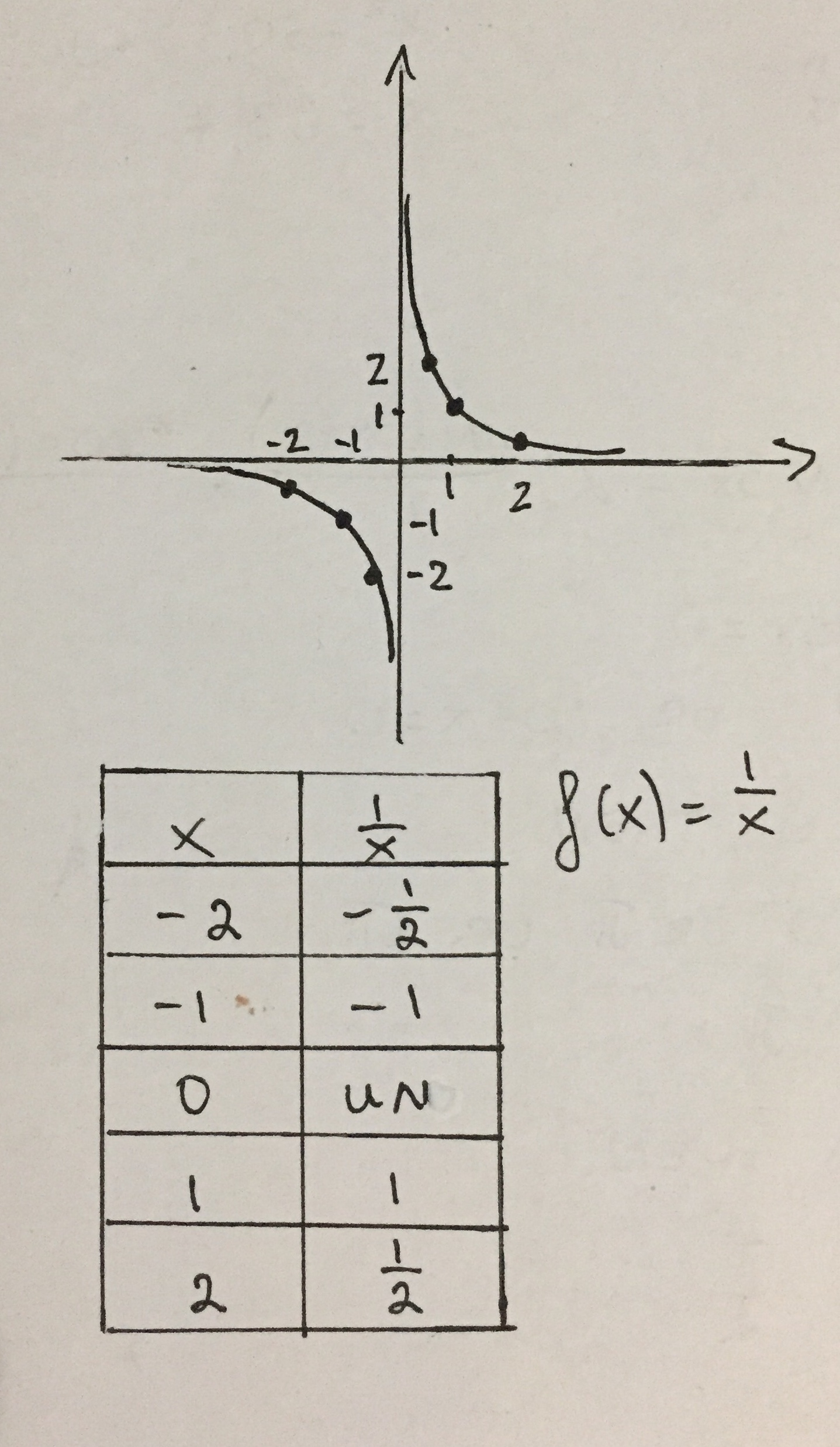Ask question

# Is the statement true or false? "The domain and the range of the reciprocal function are the set of all real numbers."# Is the statement true or false? "The domain and the range of the reciprocal function are the set of all real numbers."

Question
Analyzing functionsasked 2021-01-08
Is the statement true or false? "The domain and the range of the reciprocal function are the set of all real numbers."

## Answers (1)2021-01-09

False. The domain and range of the reciprocal function are all real numbers except the number 0 since $$\displaystyle{f{{\left({0}\right)}}}=\frac{{1}}{{0}}$$ is undefined. If x can’t be 0, y can’t be 0 either.### Relevant Questionsasked 2021-01-31
True or False. The domain of every rational function is the set of all real numbers.asked 2020-10-31
How is called the set of all images of the elements in the domain of a function?asked 2021-01-06
Determine if the statement is true or false.
For all real numbers a and b, if $$a\ <\ b\ then\ a\ <\ \frac{a\ +\ b}{2}\ <\ b.$$asked 2021-03-05
Polynomial function's f(x) coefficients are real numbers. Find the remaining zeros of f. Degree 6, zeros: i, 3 - 2i, -2 + iasked 2021-02-11
Find an nth-degree polynomial function with real coefficients satisfying the given conditions. Verify the real zeros and the given function value. n = 3, 2 and 2 - 3i are zeros, f(1) = -10asked 2020-11-03

The sampling distribution of $$\frac{S_{1}^{2}}{S_{2}^{2}}$$ is the $$\chi^2$$ distribution. Give a correct answer true or false inference for two population variances is done through their difference.asked 2021-01-04
Whether the statement “ Let A be an $$m\ \times\ n$$ matrix.
The system $$Ax = b\ \text{is consistent for all b in}\ R^{m}\ \text{if and only if the columns of A form a generating set for}\ R^{m}$$ " is true or false.asked 2020-10-27
Answer true or false to the following statements and explain your answers.
a. In multiple linear regression, we can determine whether we are extrapolating in predicting the value of the response variable for a given set of predictor variable values by determining whether each predictor variable value falls in the range of observed values of that predictor.
b. Irregularly shaped regions of the values of predictor variables are easy to detect with two-dimensional scatterplots of pairs of predictor variables, and thus it is easy to determine whether we are extrapolating when predicting the response variable.asked 2021-02-04
Find all values x = a where the function is discontinuous.
$$\displaystyle{f{{\left({x}\right)}}}={8}{x}^{{2}}+{8}{x}+{4}.$$asked 2021-01-02
Sketch the graph of the function $$\displaystyle{f{{\left({x}\right)}}}=-{x}^{{3}}+{3}{x}^{{2}}–{7}$$. List the coordinated of where extrema or points of inflection occur. State where the function is increasing or decreasing as well as where it is concave up or concave down.
...